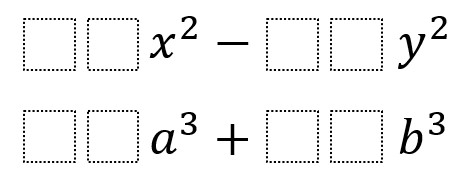# Difference of Squares and Sum of Cubes

Directions: Using the digits 0 to 9 at most one time each, place a digit in each box to make both expressions factorable.### Hint

How can we write rewrite the expressions such that (##a)^3 is equivalent to ☐☐*a^3?

If you can only find a few solutions, have you considered expressions where you first factor out the GCF?

81, 09 and 64, 27
36, 49 and 01, 27
25, 36 and 64, 01

There are a lot (not infinite) of solutions once you include taking out the GCF.

For example:
36x^2 – 09y^2 –> 9(4x^2 -1y^2) –> factorable!
and
81a^3 + 24b^3 –> 3(27a^3 + 8b^3) –> factorable!

Source: Jack Assaf

### Check Also

Directions: Directions: Using the integers from −9 to 9 at most once each, place one …

### One comment

1.Paul Muckerheide

A question might be what does factorable mean z
01x^2-23y^2=
(x+y*23^.5)(x-y*23^.5) if it meets the definition of factorable.
Question is can we factor
x^2-i^2?
There is some importance to consider this topic since an approach to solving all quadratics is to slide function so axis of symmetry and the linear coefficient always becomes 0 meaning solutions ( real and non real) can be found by factoring with difference of squares pattern. Set linear factors to zero and solve.